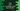# Java program to print a rectangle using any special character## Java tutorial program to print a rectangle using special character like star, dollar etc :

In this tutorial, we will learn how to print a rectangle in Java using any special character . For example take the below rectangle :



The height of this rectangle is 7 and width is 6. Also, we are using ’$' to print the rectangle. Instead of '$’, we can use any character to print.

Algorithm we are going to use in this example is as below :

### Algorithm :

1. Take the height and width of the rectangle from the user.
2. Also, take the character that the user wants to print the rectangle.
3. Run one ‘for’ loop. This will run same time as the height of the rectangle.
4. Each run of this ‘for’ loop, run one inner loop. This inner loop will run same as its width.
5. For the first run of the outer loop, print character as the width of the inner loop. Because , this will be the first row of the rectangle.
6. For the second to (height -1) run of the outer loop, print only the first and the last element for that row.
7. For the last run of the outer loop, print characters same as first run. Because the last row will also contain full row of characters.

Let’s take a look into the example program below for better understanding :

### Java Program :

import java.util.Scanner;

public class Main {
/**
* Utility function to print
*/
private static void println(String str) {
System.out.println(str);
}

private static void print(String str) {
System.out.print(str);
}

private static void printRectangle(int height, int width, String c) {
for (int i = 0; i < height; i++) {
if (i == 0 || i == height - 1) {
//for first line and last line , print the full line
for (int j = 0; j < width; j++) {
print(c);
}
println(""); //enter a new line
} else {
//else
for (int j = 0; j < width; j++) {
if (j == 0 || j == width - 1) {
//print only the first and last element as the character
print(c);
} else {
//else print only blank space for the inner elements
print(" ");
}
}
println(""); //enter a new line
}
}
}

public static void main(String[] args) {
Scanner scanner = new Scanner(System.in);

print("Enter the height of the rectangle : ");
int height = scanner.nextInt();

print("Enter the width of the rectangle : ");
int width = scanner.nextInt();

print("Enter the character you want to print the rectangle : ");
String c = scanner.next();

printRectangle(height, width, c);
}

}

### Sample Output :

Enter the height of the rectangle : 7
Enter the width of the rectangle : 6
Enter the character you want to print the rectangle : \$